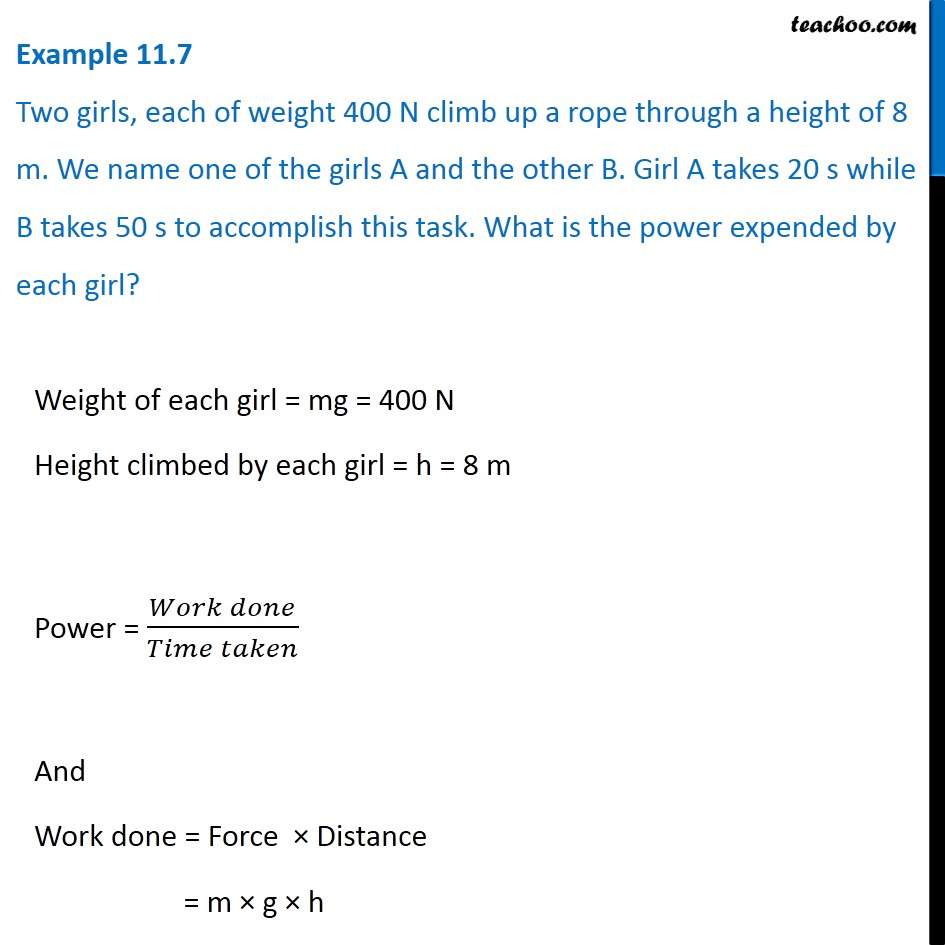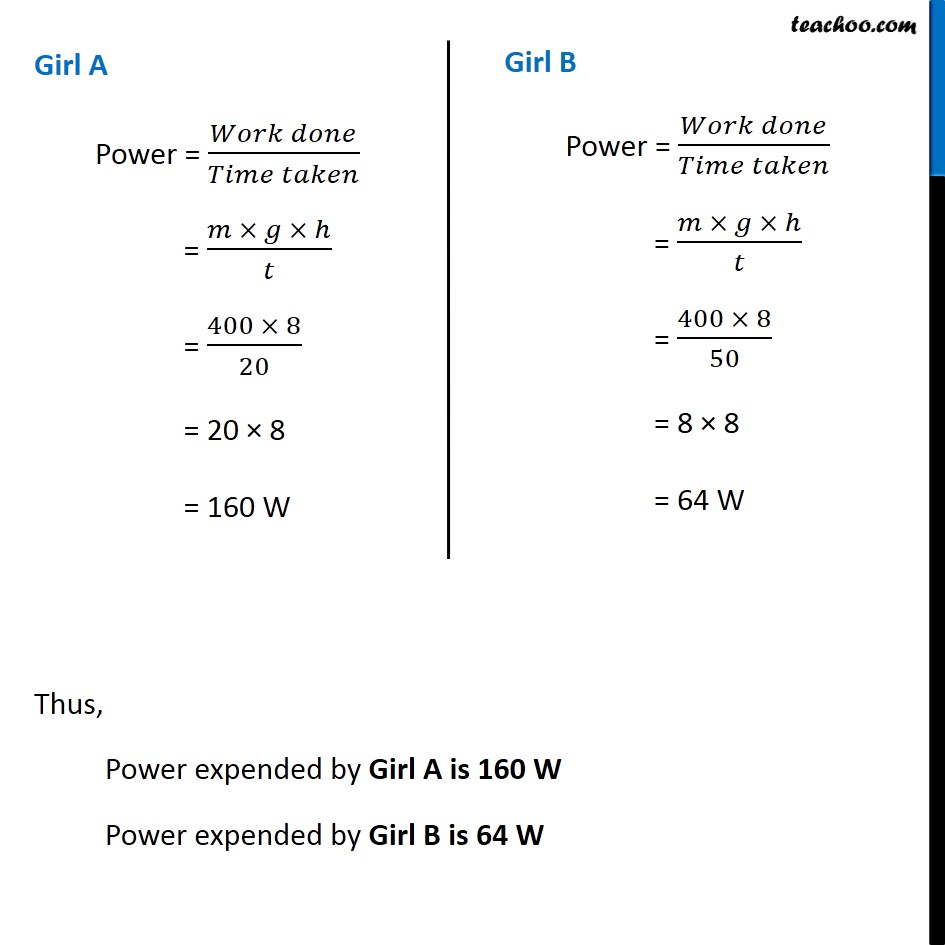Examples from NCERT Book

Class 9
Chapter 11 Class 9 - Work and EnergyLearn in your speed, with individual attention - Teachoo Maths 1-on-1 Class

### Transcript

Example 11.7 Two girls, each of weight 400 N climb up a rope through a height of 8 m. We name one of the girls A and the other B. Girl A takes 20 s while B takes 50 s to accomplish this task. What is the power expended by each girl? Weight of each girl = mg = 400 N Height climbed by each girl = h = 8 m Power = (𝑊𝑜𝑟𝑘 𝑑𝑜𝑛𝑒)/(𝑇𝑖𝑚𝑒 𝑡𝑎𝑘𝑒𝑛) And Work done = Force × Distance = m × g × h Girl A Power = (𝑊𝑜𝑟𝑘 𝑑𝑜𝑛𝑒)/(𝑇𝑖𝑚𝑒 𝑡𝑎𝑘𝑒𝑛) = (𝑚 × 𝑔 × ℎ)/𝑡 = (400 × 8)/20 = 20 × 8 = 160 W Girl B Power = (𝑊𝑜𝑟𝑘 𝑑𝑜𝑛𝑒)/(𝑇𝑖𝑚𝑒 𝑡𝑎𝑘𝑒𝑛) = (𝑚 × 𝑔 × ℎ)/𝑡 = (400 × 8)/50 = 8 × 8 = 64 W Thus, Power expended by Girl A is 160 W Power expended by Girl B is 64 W